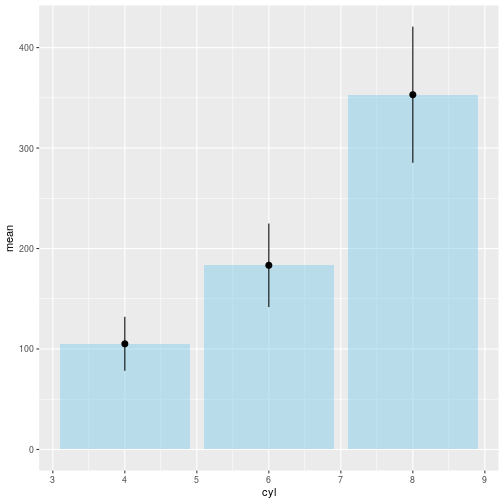Errorbars on barcharts

Errorbars

An errorbar is a graphical indication of the standard deviation of a measurement. Or a confidence interval.

The mean of a measurement is something. We want to illustrate how confident we are of that value.

How do we plot that?

I am going to use three libraries:

library(ggplot2)
library(dplyr)
library(tibble)

ggplot2 for plotting, dplyr to make manipulating data easier. And tibble to support a single line later.

Then, we need some data.

data <- data.frame(
name=letters[1:3],
mean=sample(seq(4,15),3),
sd=c(1,0.2,3)
)

I now have a dataframe with three variables, name, mean and standard deviation (sd).

How do we plot that?

Simple:

data %>%
ggplot() +
geom_bar( aes(x=name, y=mean), stat="identity", fill="skyblue") +
geom_errorbar(aes(x=name, ymin=mean-sd, ymax = mean+sd), width=0.1)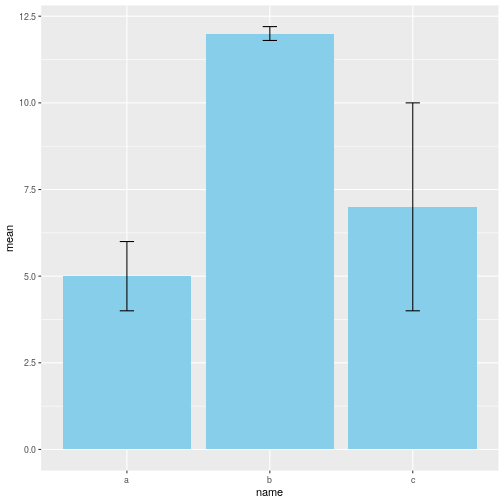So. What on earth was that?

The pipe-operator %>% takes whatever is on the left-hand-side, and inserts it as the first variable in whatever is on the right-hand-side.

What is on the right-hand-side is the ggplot function. That would normally have a datat=something as the first variable. Here that is the data we constructed earlier.

To that initial plot, which is completely empty, we add a geom_bar. That plots the bars on the plot. It takes an x-value, name, and a y-value, mean. And we tell the function, that rather than counting the number of observations of each x-value (the default behavior of geom_bar), it should use the y-values provided. We also want a nice lightblue color for the bars.

To that bar-chart, we now add errorbars. geom_errorbar needs to know the x- and y-values of the bars, in order to place them correctly. It also needs to know where to place the upper errorbar, and the lower errorbar. And we supply the information that ymin, the lower, should be the mean value minus the standard deviation. And the upper bar, ymax, the sum of the mean and sd. Finally we need to decide how broad those lines should be. We do that by writing “width=0.1”. We do not actually have to, but the default value results in an ugly plot.

And there you go, a barchart with errorbars!

Next step

That was all very nice. However! We do not usually have a nice dataframe with means and standard deviations calculated directly. More often, we have a dataframe like this:

mtcars %>%
remove_rownames() %>%
select(cyl, disp) %>%
##   cyl disp
## 1   6  160
## 2   6  160
## 3   4  108
## 4   6  258
## 5   8  360
## 6   6  225

I’ll get back to what the code actually means later.

Here we have 32 observations (only 6 shown above), of the variables “cyl” and “disp”. I would now like to make a barplot of the mean value of disp for each of the three different values or groups in cyl (4,6 and 8). And add the errorbars.

You could scroll through all the data, sort them by cyl, manually count the number of observations in each group, add the disp, divide etc etc etc.

But there is a simpler way:

mtcars %>%
remove_rownames() %>%
select(cyl, disp) %>%
group_by(cyl) %>%
summarise(mean=mean(disp), sd=sd(disp))
## # A tibble: 3 x 3
##     cyl  mean    sd
##   <dbl> <dbl> <dbl>
## 1     4  105.  26.9
## 2     6  183.  41.6
## 3     8  353.  67.8

mtcars is a build-in dataset (cars in the us in 1974). I send that, using the pipe-operator, to the function remove_rownames, that does exactly that. We don’t need them, and they will just confuse us. That result is then send to the function select, that selects the two columns/variables cyl and disp, and discards the rest. Next, we group the data according to the value of cyl. There are three different values, 4, 6 and 8. And then we use the summarise function, to calculate the mean and the standard deviation of disp, for each of the three groups.

Now we should be ready to plot. We just send the result above to the plot function from before:

mtcars %>%
remove_rownames() %>%
select(cyl, disp) %>%
group_by(cyl) %>%
summarise(mean=mean(disp), sd=sd(disp)) %>%
ggplot() +
geom_bar( aes(x=cyl, y=mean), stat="identity", fill="skyblue") +
geom_errorbar(aes(x=cyl, ymin=mean-sd, ymax = mean+sd), width=0.1)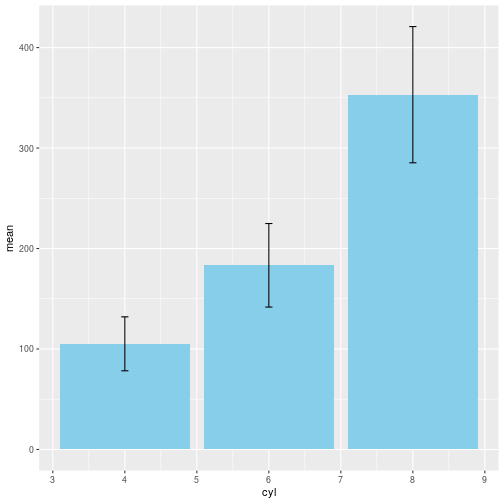All we need to remember is to change “name” in the original to “cyl”. All done!

But wait! There is more!!

Those errorbars can be shown in more than one way.

Let us start by saving our means and sds in a dataframe:

data <- mtcars %>%
remove_rownames() %>%
select(cyl, disp) %>%
group_by(cyl) %>%
summarise(mean=mean(disp), sd=sd(disp))

geom_crossbar results in this:

data %>%
ggplot() +
geom_bar( aes(x=cyl, y=mean), stat="identity", fill="skyblue") +
geom_crossbar( aes(x=cyl, y=mean, ymin=mean-sd, ymax=mean+sd))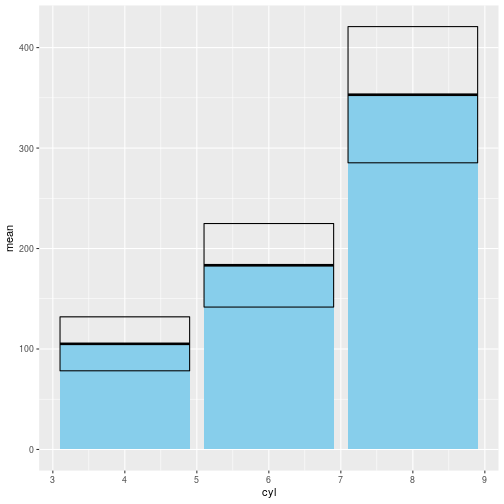I think it is ugly. But whatever floats your boat.

Then there is just a vertival bar, geom_linerange. I think it makes it a bit more difficult to compare the errorbars. On the other hand, it results in a plot that is a bit more clean:

data %>% ggplot() +
geom_bar( aes(x=cyl, y=mean), stat="identity", fill="skyblue") +
geom_linerange( aes(x=cyl, ymin=mean-sd, ymax=mean+sd))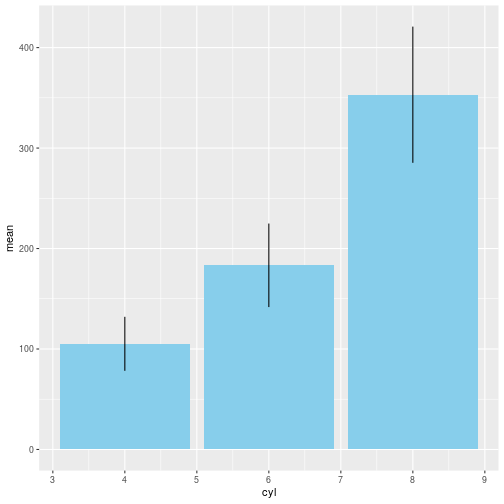And here is geom_pointrange. The mean is shown as a point. This probably works best without the bars.

data %>% ggplot() +
geom_bar( aes(x=cyl, y=mean), stat="identity", fill="skyblue", alpha=0.5) +
geom_pointrange( aes(x=cyl, y=mean, ymin=mean-sd, ymax=mean+sd))